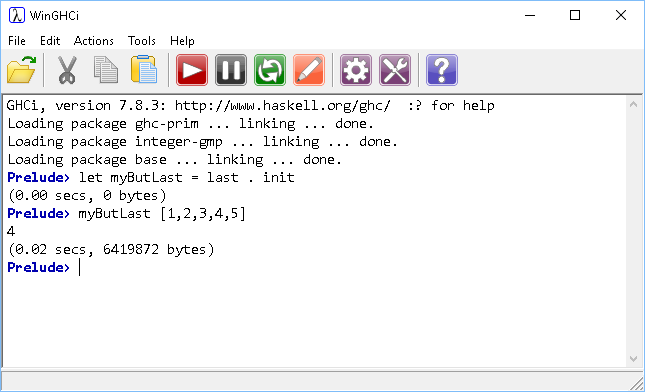# Introduction To Haskell Last But One

## Introduction

Problem 2 of the 99 challenges us to find the last but one element of a list.

The simplest way to do this is to use the last and init functions. The init function returns a list consisting of everything except the last item. The last item of that new list will be the second to last item of the first list.

```myButLast = last . init
```

We can compose these two functions together and get a very simple solution to the problem,This is a little too simple though. Let's assume that we don't have those two functions and that we want to avoid the following,Here is a better version,

```myButLast [] = error "List Empty"
myButLast [x] = error "Only one item"
myButLast (x:xs)
| length xs>2 = myButLast.tail \$ xs
| length xs==2 = head xs
```

We have multiple definitions of the function here, each one catering for a different scenario. Let's look at them ony at a time. The first definition accepts an empty list as an argument and returns an error message. The second definition is for a list of length 1. Such a list has no last but one element - an error is returned for this too.

The last definition is the interesting one. The argument is described using pattern matching. Using this notation, x represents the head of the list, xs represents the tail. The first guard states that if the tail of the list (xs) has more than two items, the function is called again with the tail of xs as the argument. In this line, the dollar sign is the application operator. This forces the interpreter to evaluate the tail function before using it as an argument. THe final guard is the stopping condition for this function. When the tail of the argument consists of two items, the first of those two will be the result we want.

In summary, this function takes the original list and divides into its head (first item) and tail (the other items). If the tail consists of two items, return the first. If the tail is longer than two items, repeat the process with everything but the first item in the tail. It chops the first item from the list until we are left with only two items, the first of these is the last but one.

## Challenges

1. Last but three.
2. Last but n.